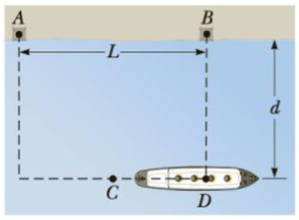Chapter 14, Problem 39P

Chapter
Section
Textbook Problem

The ship in Figure P14.39 travels along a straight line parallel to the shore and a distance d = 600 m from it. The ship’s radio receives simultaneous signals of the same frequency from antennas A and B, separated by a distance L = 800 m. The signals interfere constructively at point C, which is equidistant from A and B. The signal goes through the first minimum at point D, which is directly outward from the shore from point B. Determine the wave-length of the radio waves.Figure P14.39

To determine
The wave length of the radio waves.

Explanation

Given Info: The distance of the ship from the shore is 600 m and the points A and B are separated by a distance of 800 m.

Formula for to calculate the distance between point A and D is,

lAD is the distance between point A and D,

L is the distance of separation between A and B,

d is the distance of the ship from the shore,

Substitute 600m for d and 800m for AB in the above expression to get lAD .

The distance between the point A and D is 1000 m

Still sussing out bartleby?

Check out a sample textbook solution.

See a sample solution

The Solution to Your Study Problems

Bartleby provides explanations to thousands of textbook problems written by our experts, many with advanced degrees!

Get Started

In this nation, stringent controls make it difficult to obtain a bogus nutrition credential. T F

Nutrition: Concepts and Controversies - Standalone book (MindTap Course List)

How does astronomy help answer the question “What are we?”

Horizons: Exploring the Universe (MindTap Course List)

Which amino acids could be referred to as derivatives of butanoic acid?

Chemistry for Today: General, Organic, and Biochemistry

The dinosaurs died out ______ million years ago.

Biology: The Unity and Diversity of Life (MindTap Course List)